# ML Aggarwal Solutions for Class 9 Maths Chapter 11: Mid Point Theorem

ML Aggarwal Solutions for Class 9 Maths Chapter 11 Mid Point Theorem is an important resource for students of ICSE board. The solutions help students obtain a clear idea about the sort of questions that would be asked in the exam and the method of answering them. The experts at BYJU’S provide ML Aggarwal Solutions for Class 9 Maths Chapter 11 Mid Point Theorem PDF to help students with their exam preparation.

Chapter 11 has problems on triangles which can be solved by using the Mid Point Theorem.The PDF of ML Aggarwal Solutions can be referred by students to clear their doubts during the self study process. The main aim of providing solutions chapter wise is to help students with their exam preparation, based on the current syllabus of ICSE board.

## ML Aggarwal Solutions for Class 9 Maths Chapter 11: Mid Point Theorem Download PDF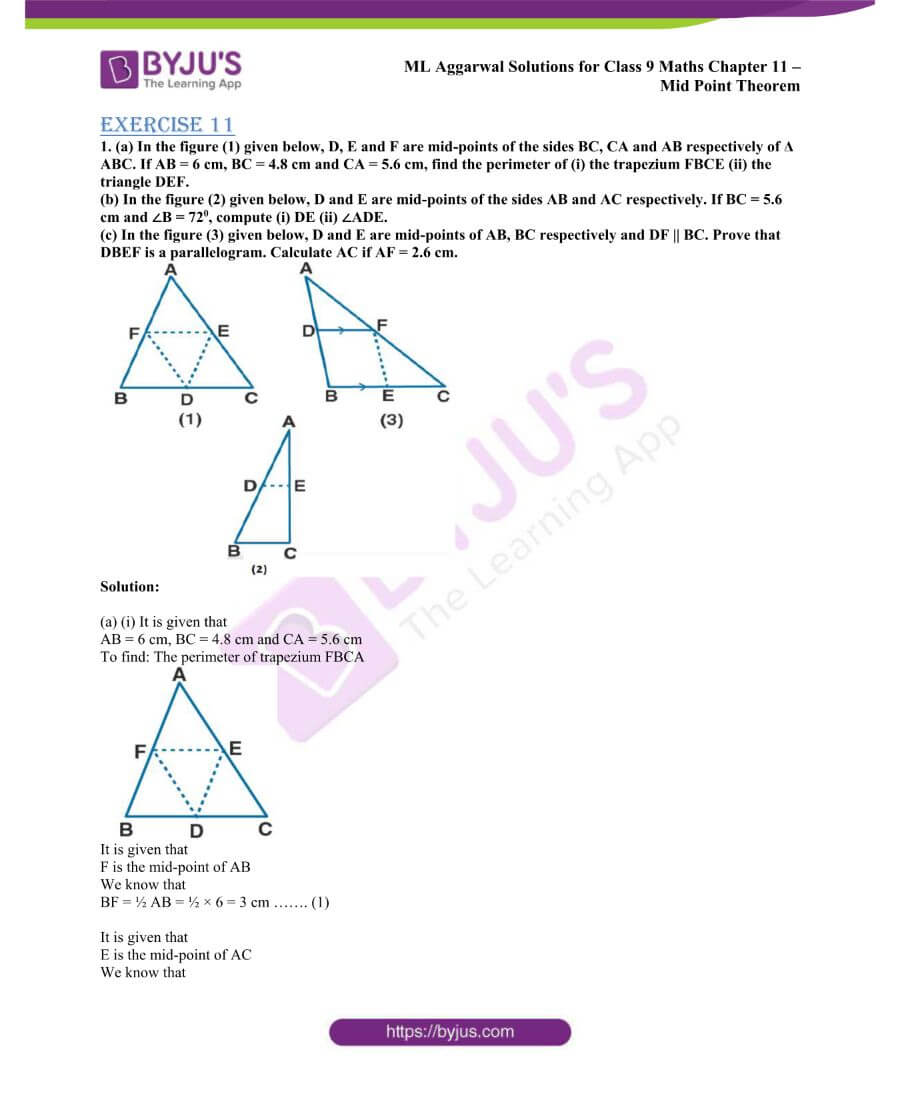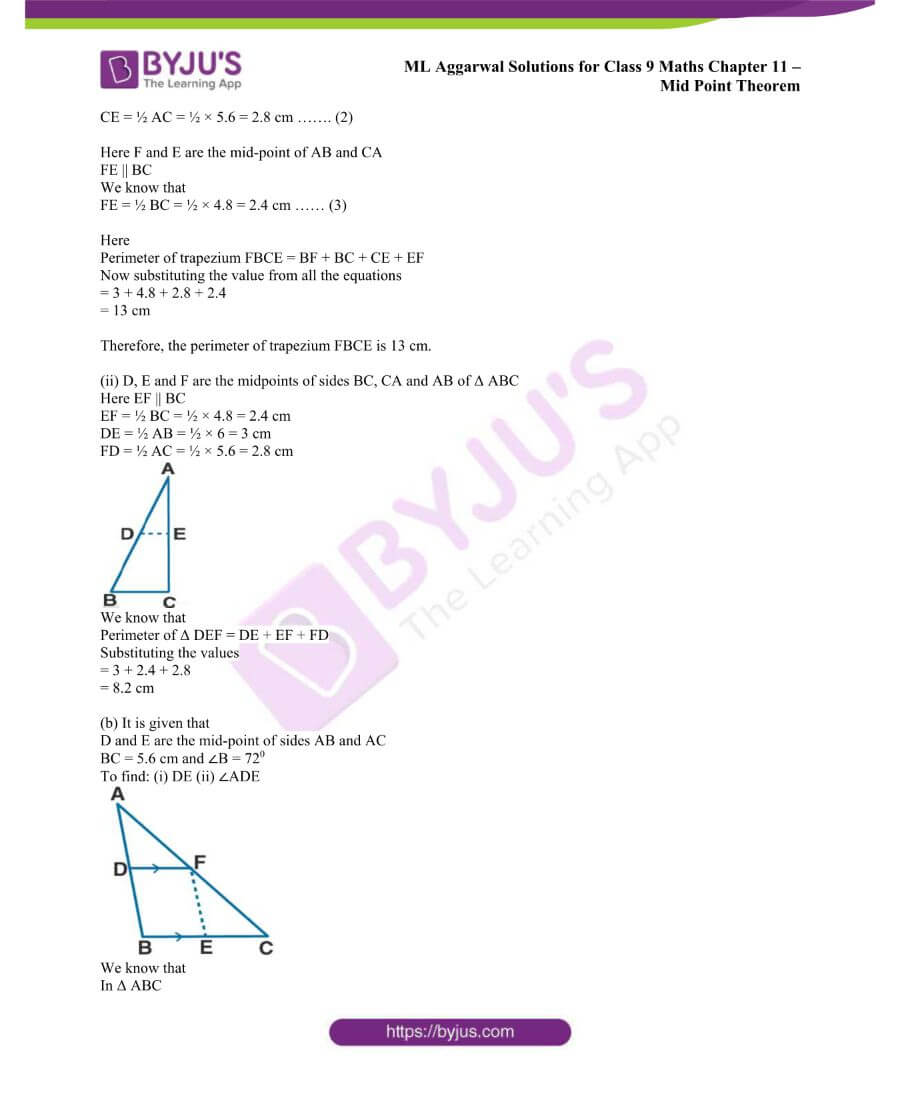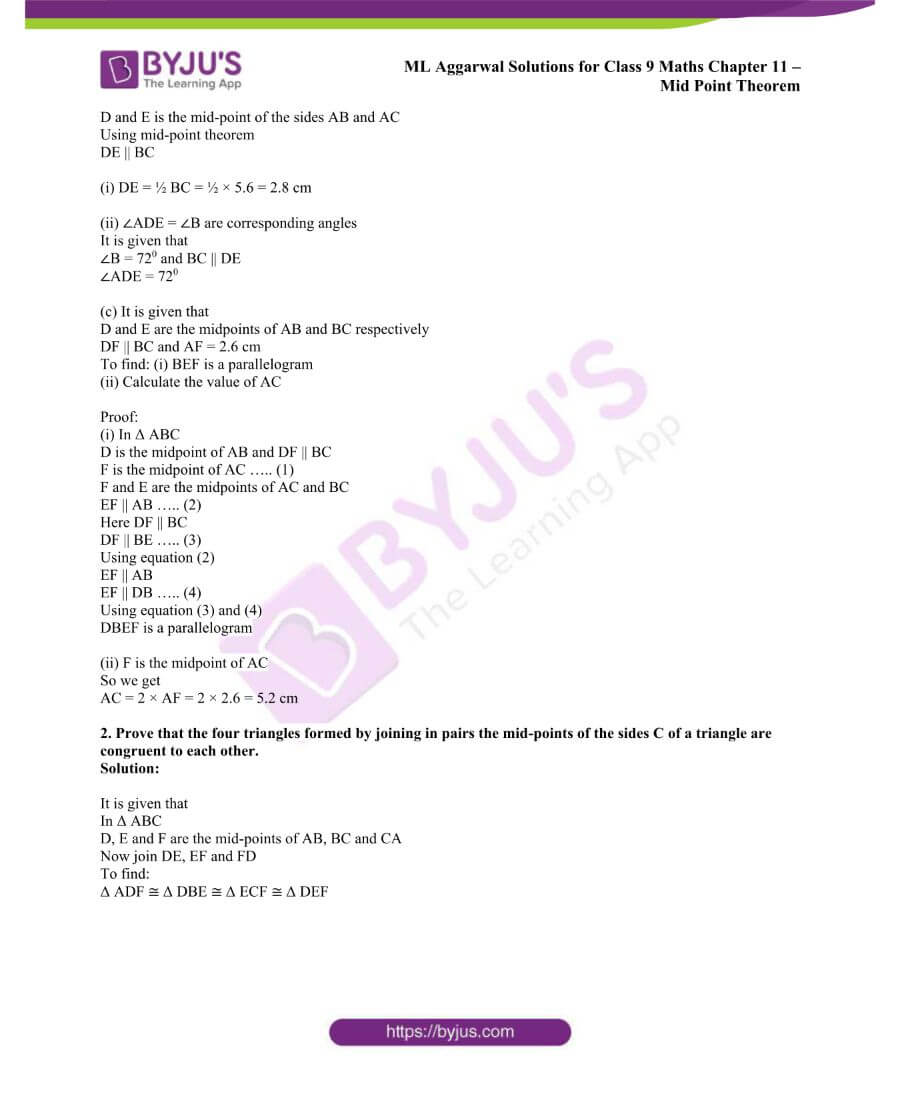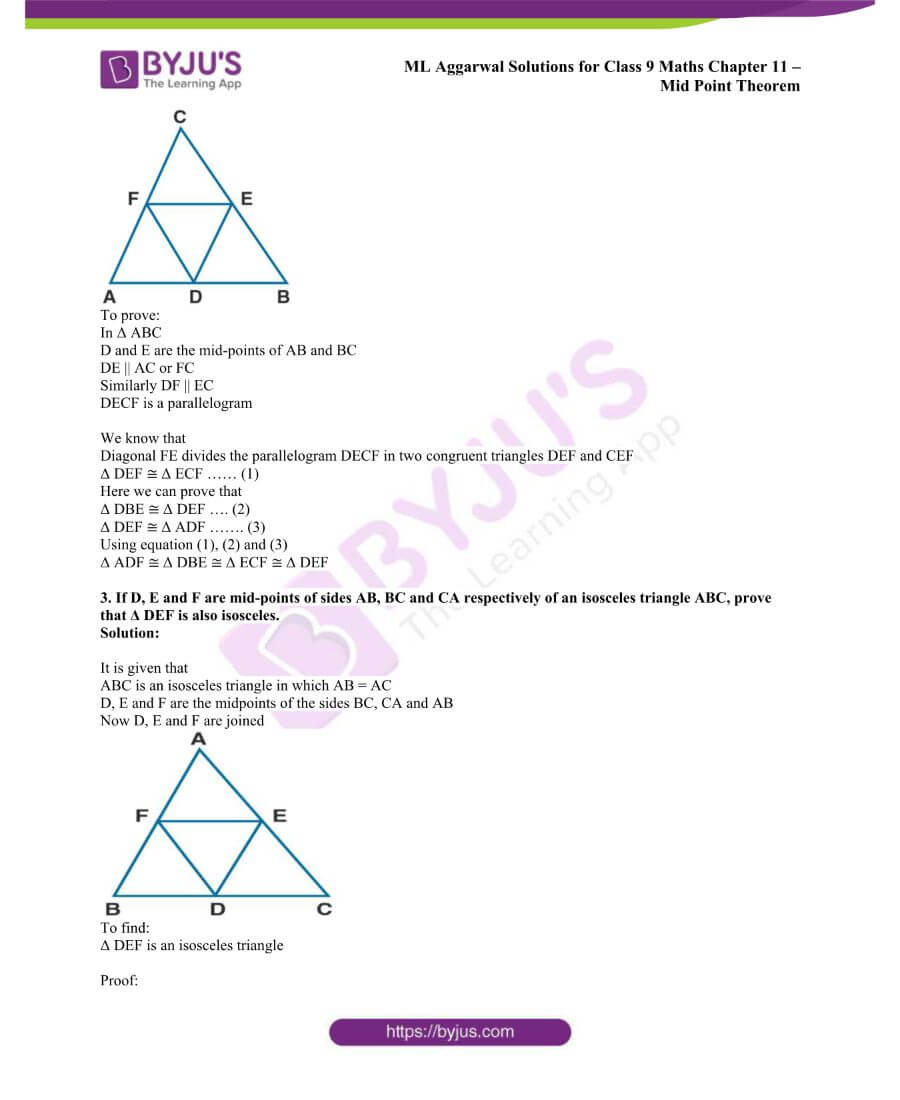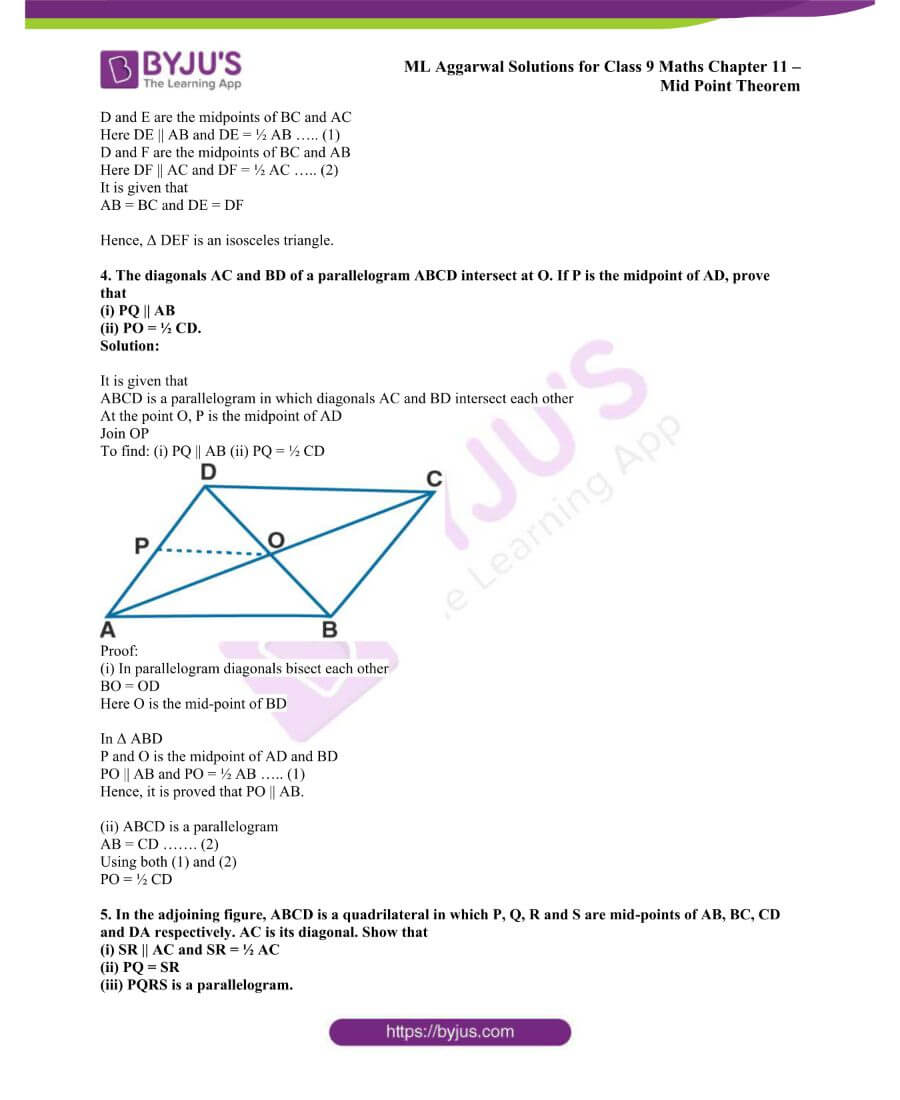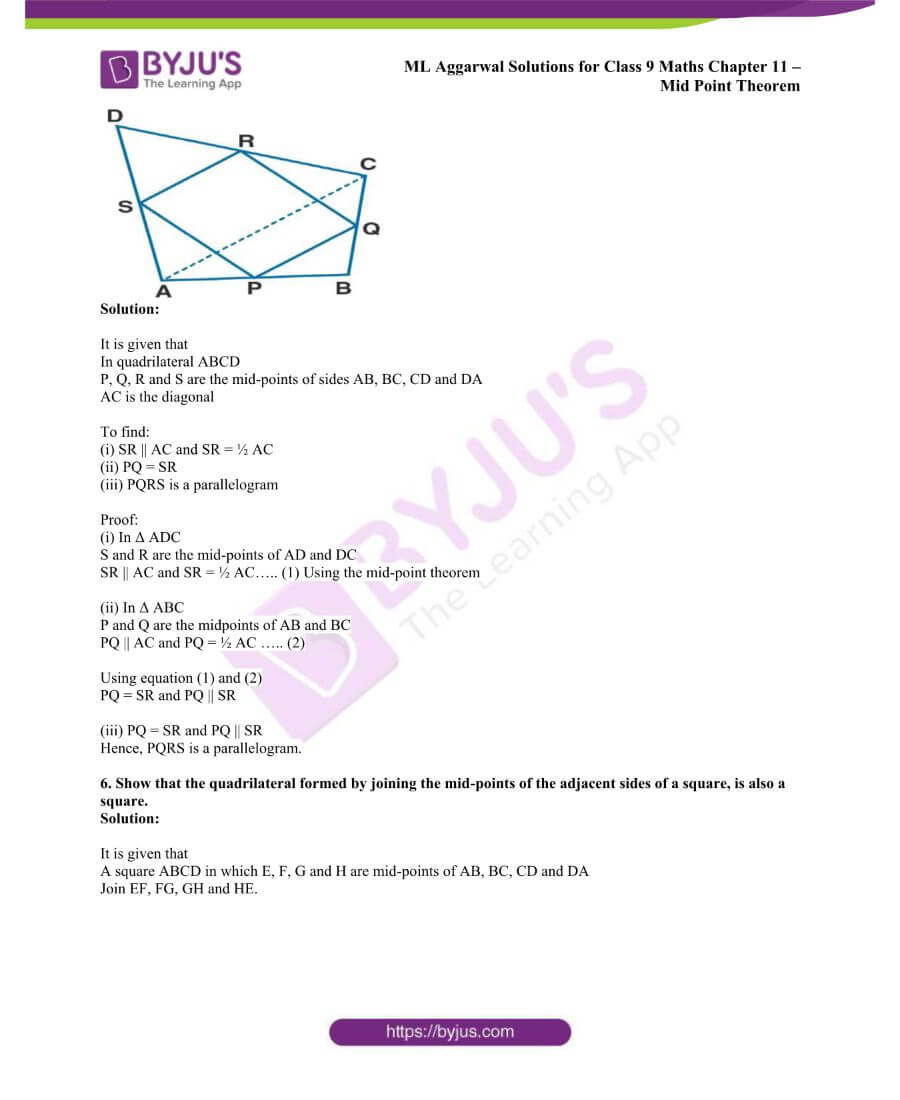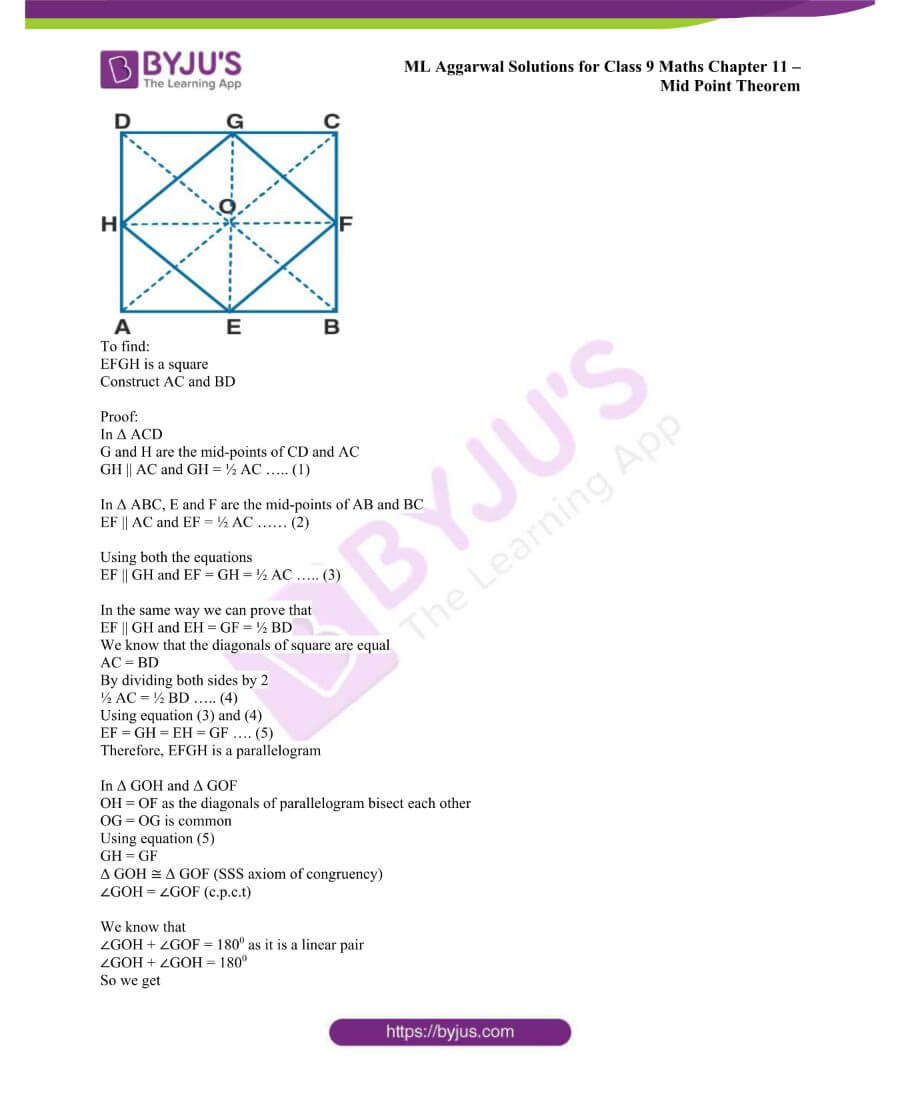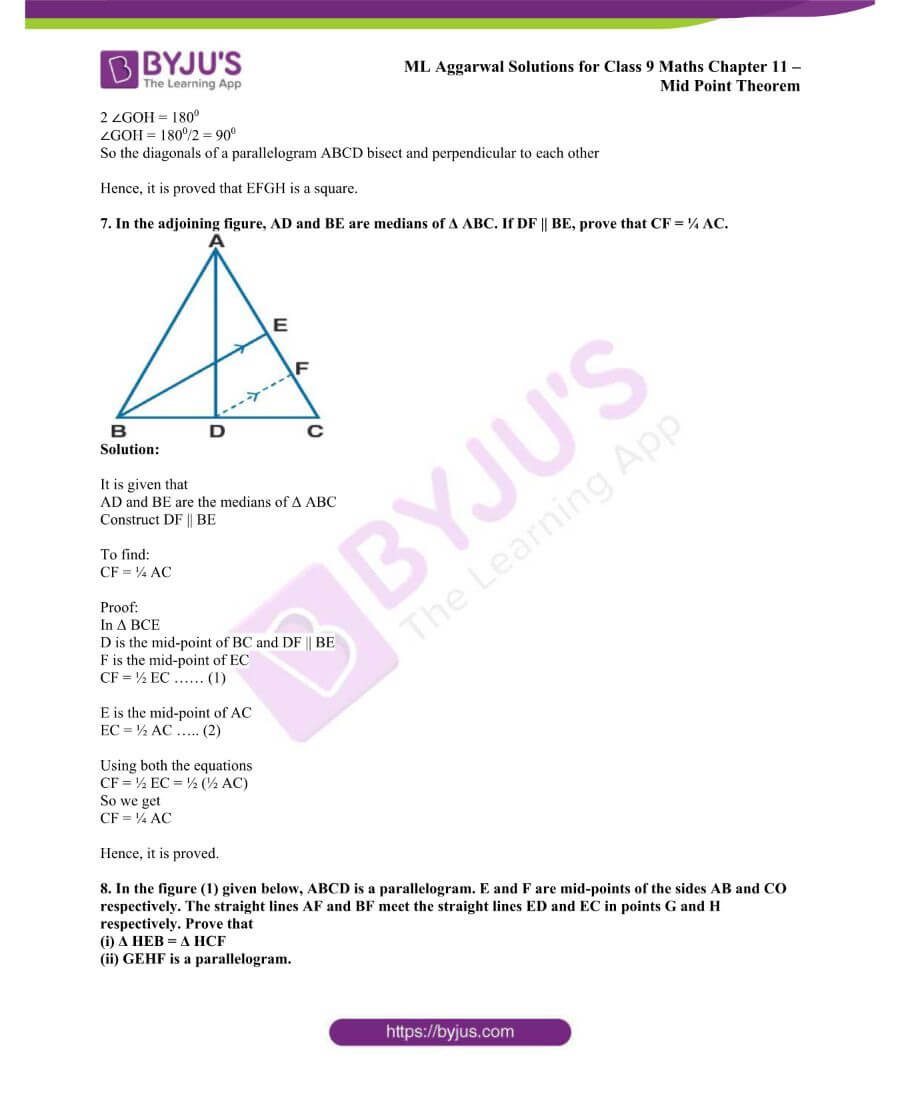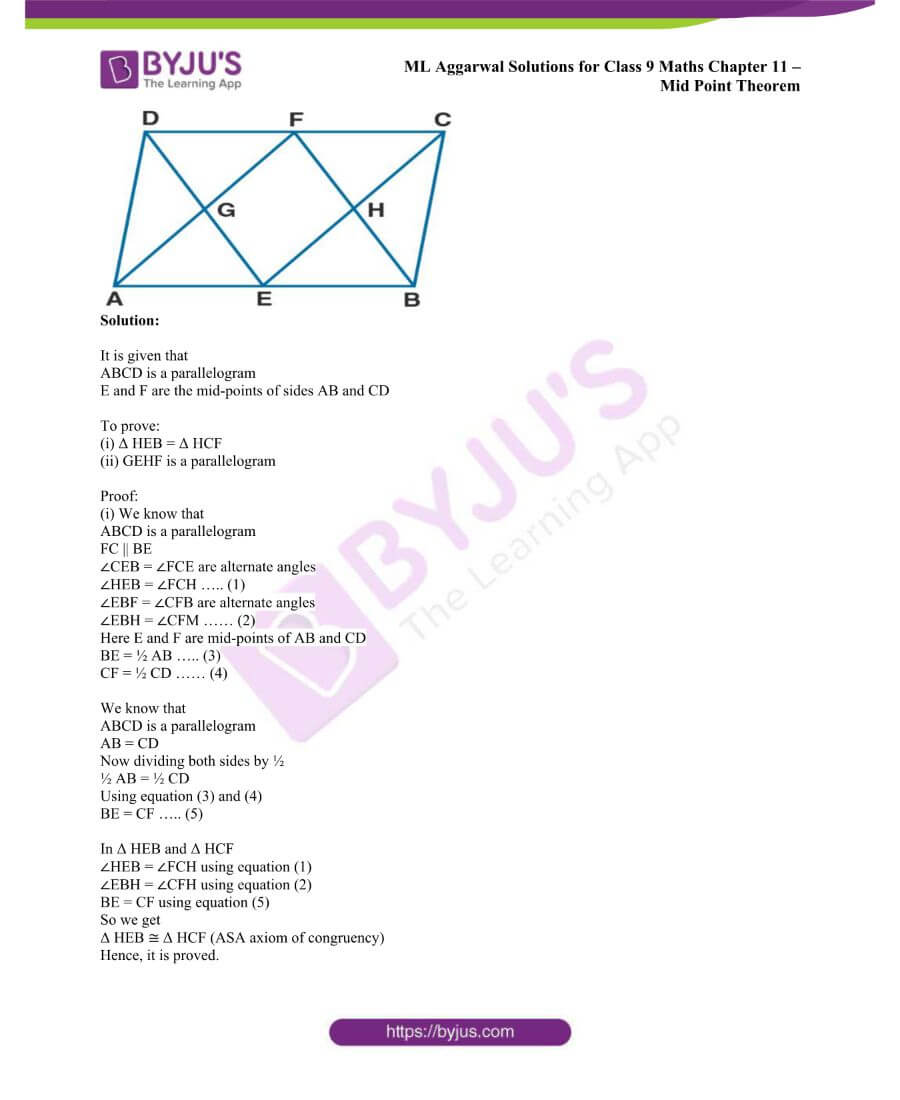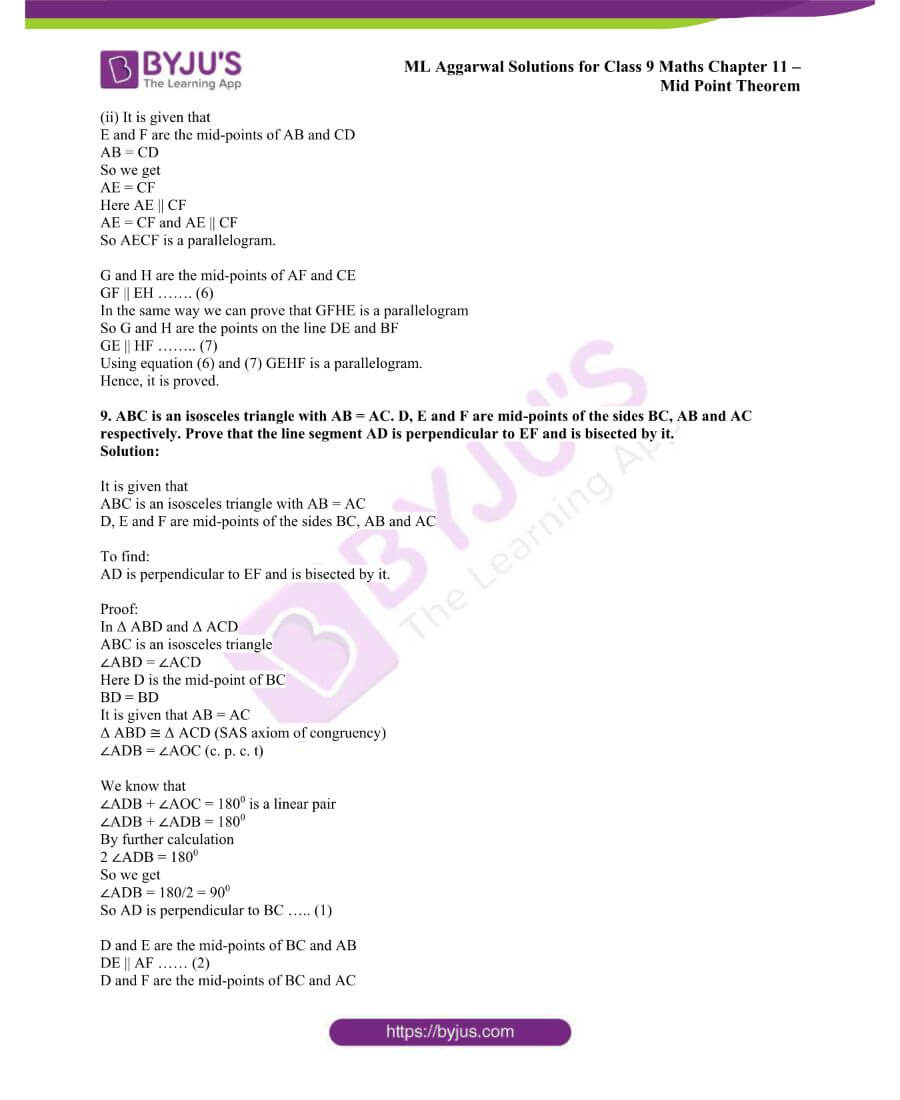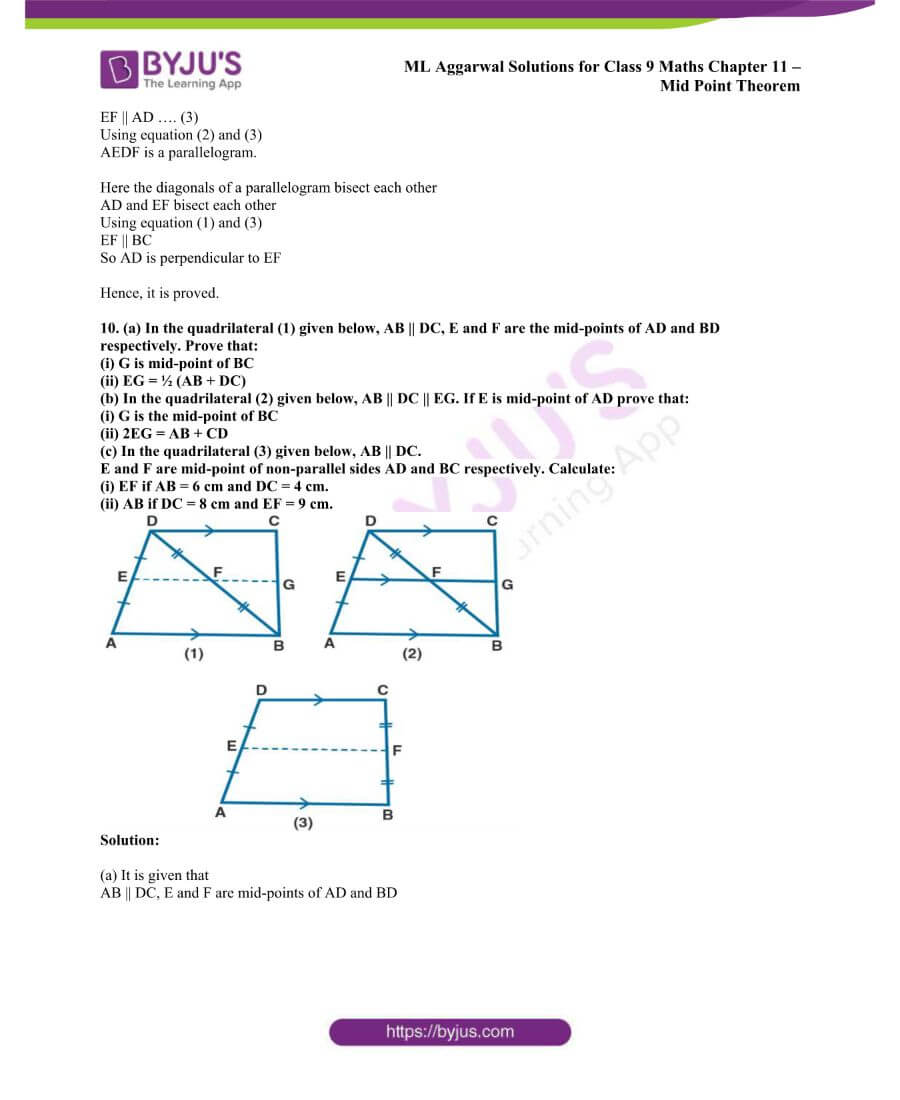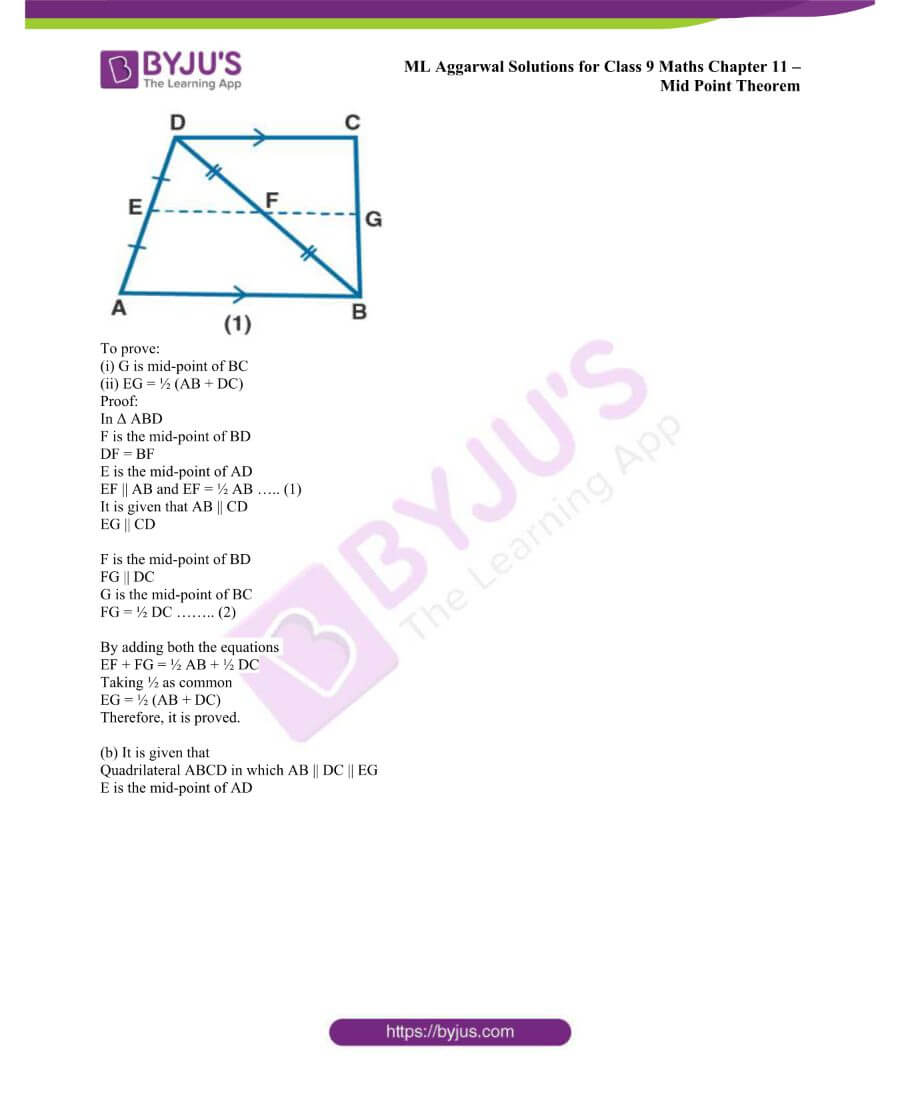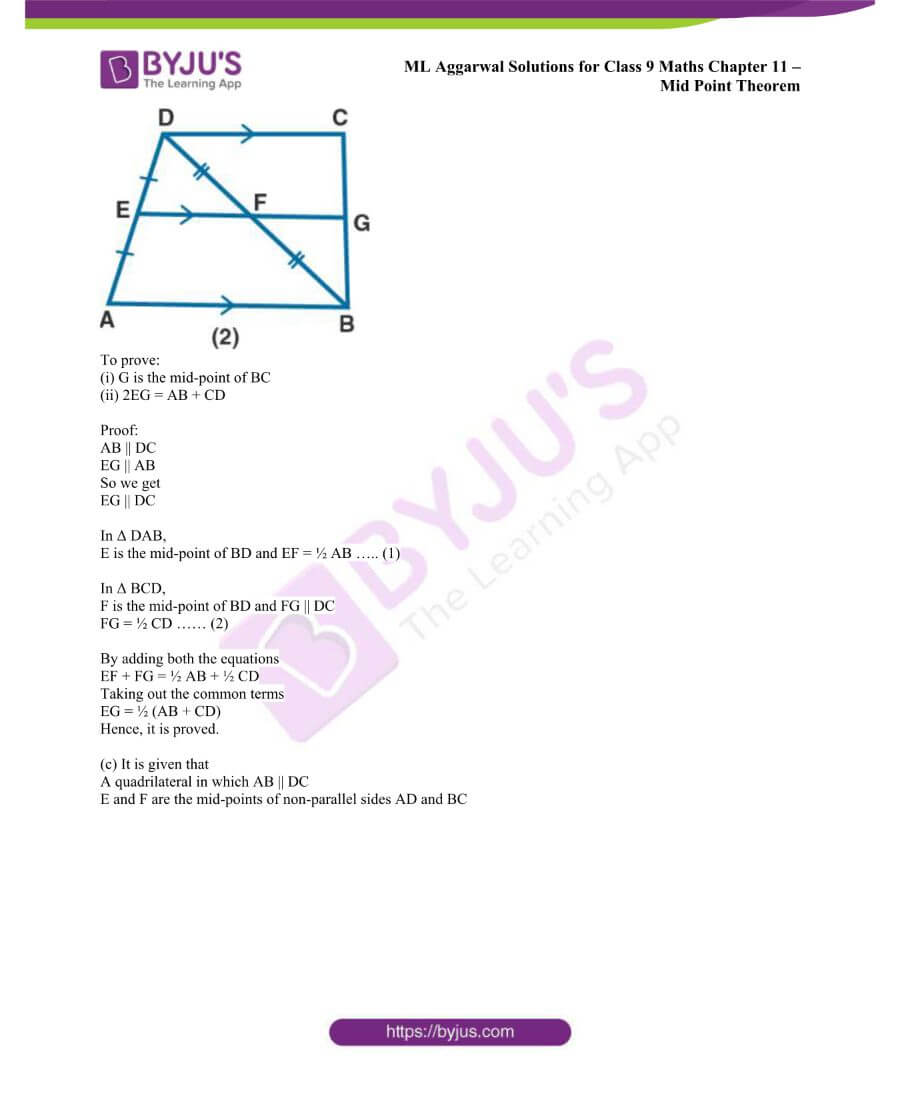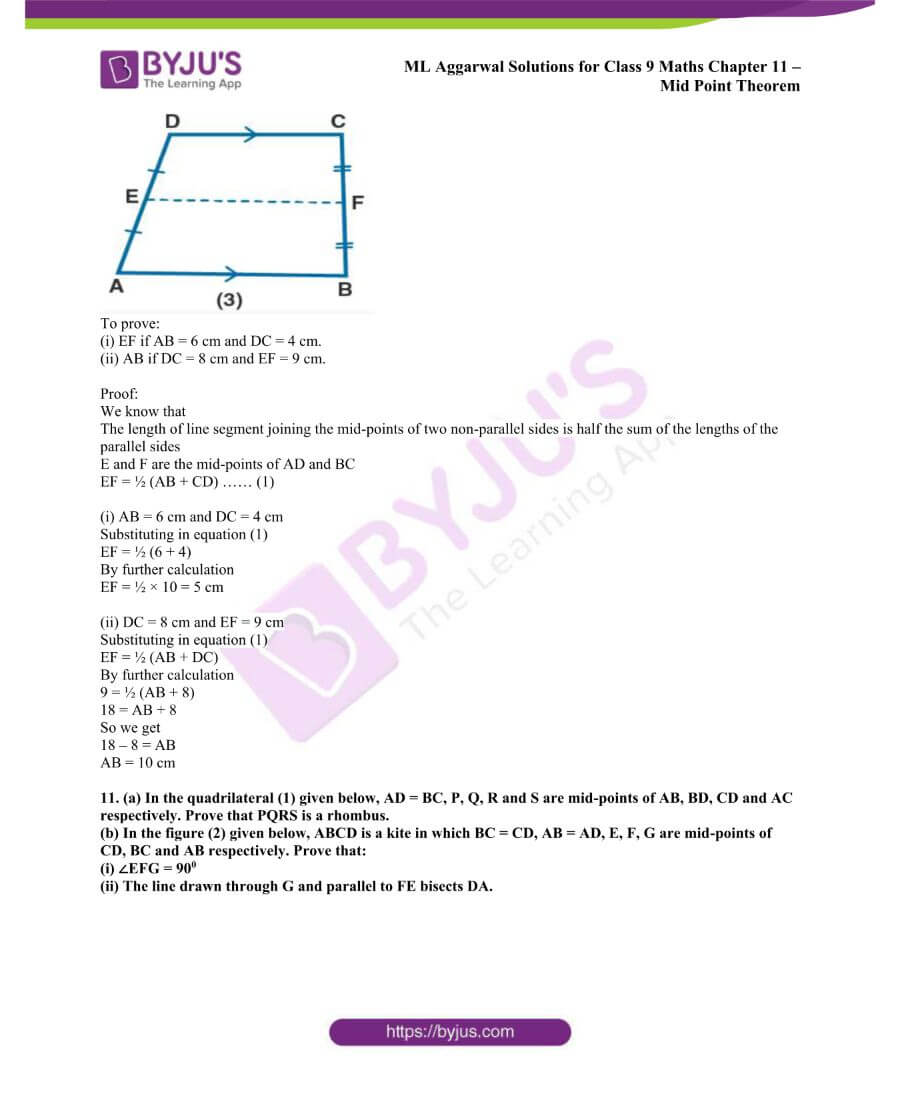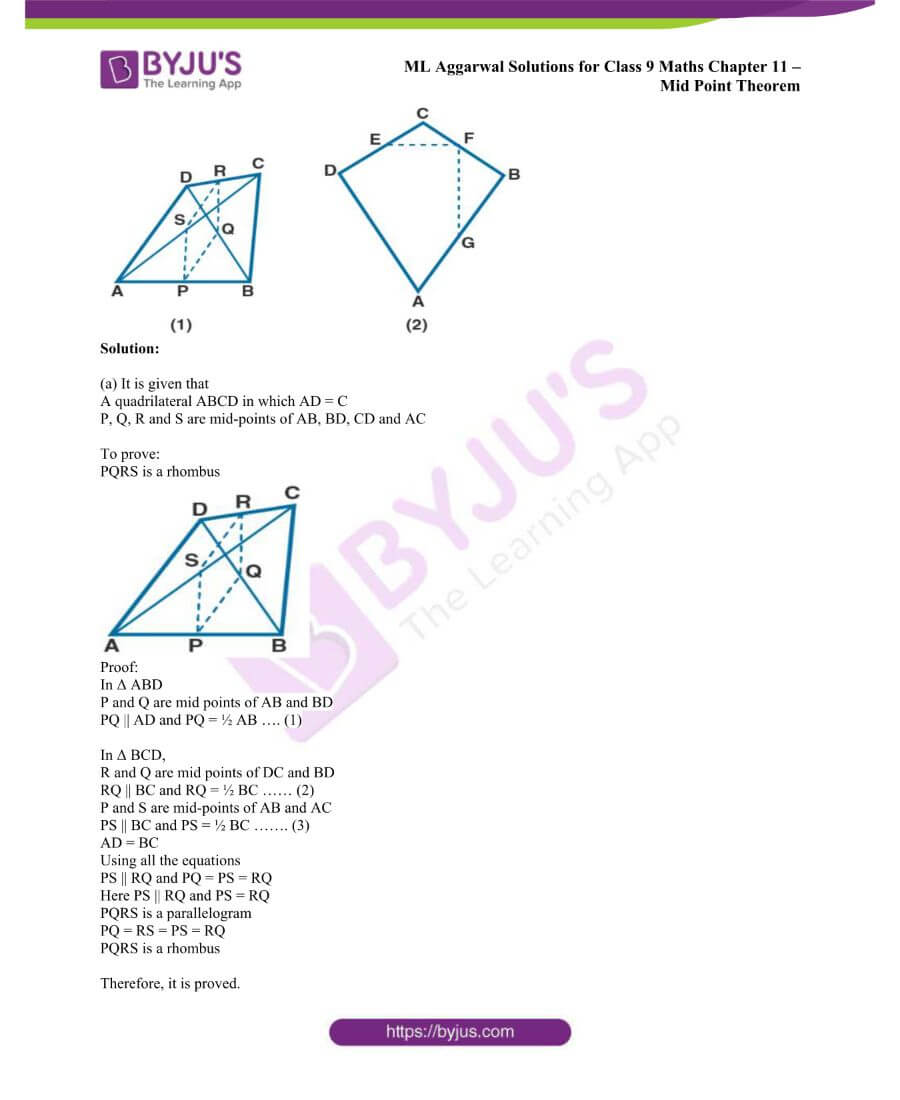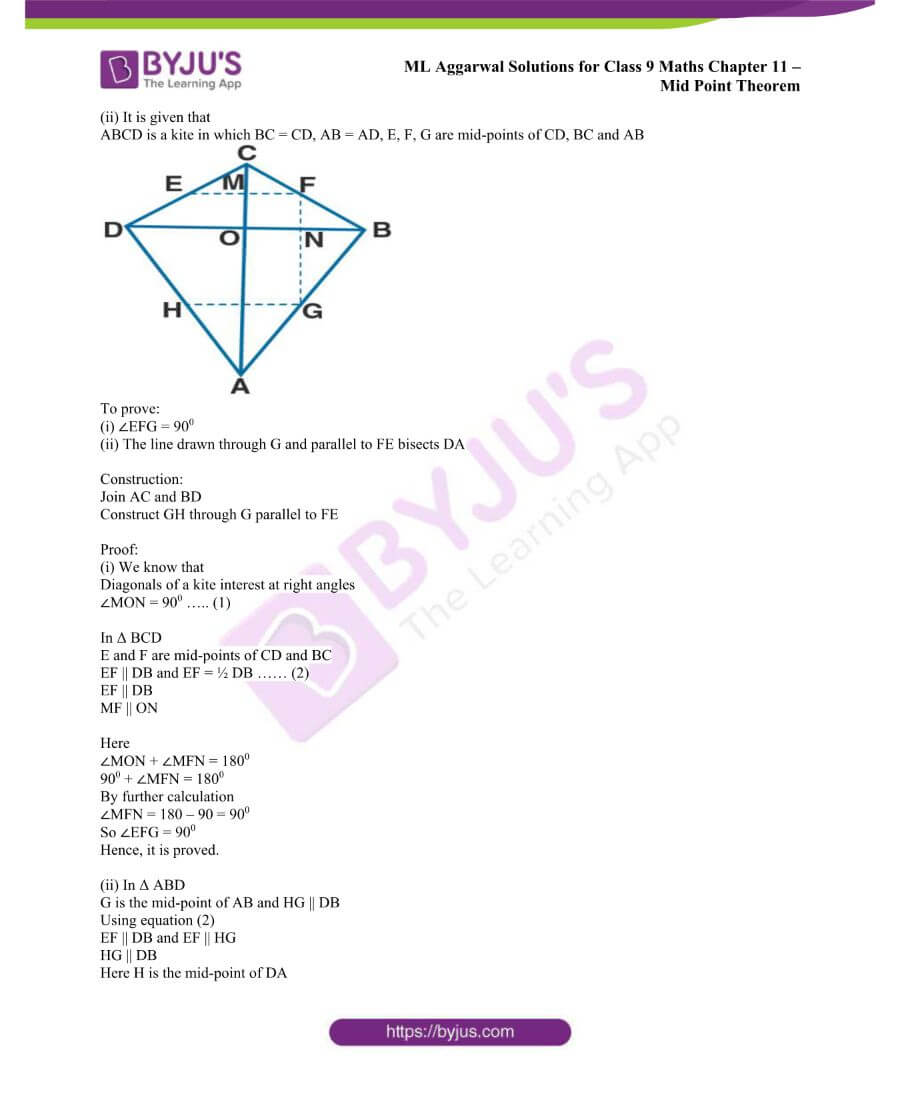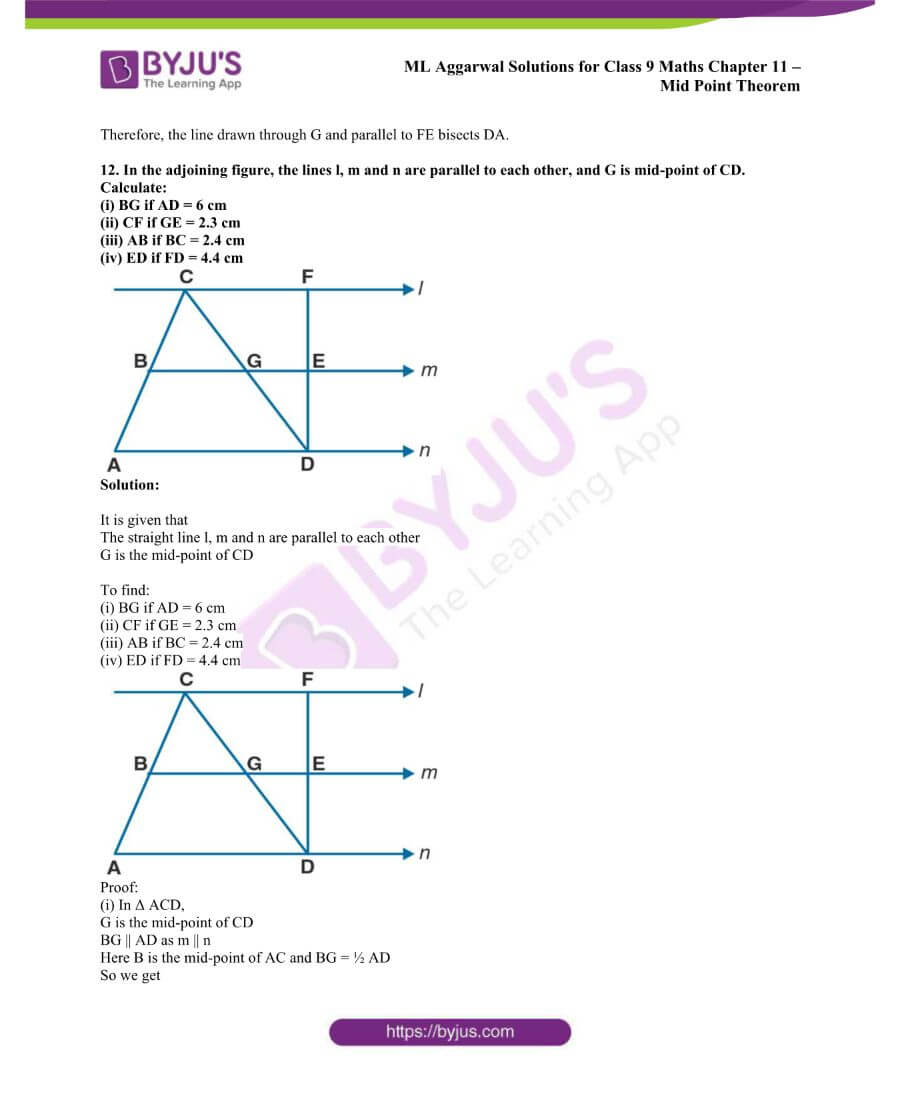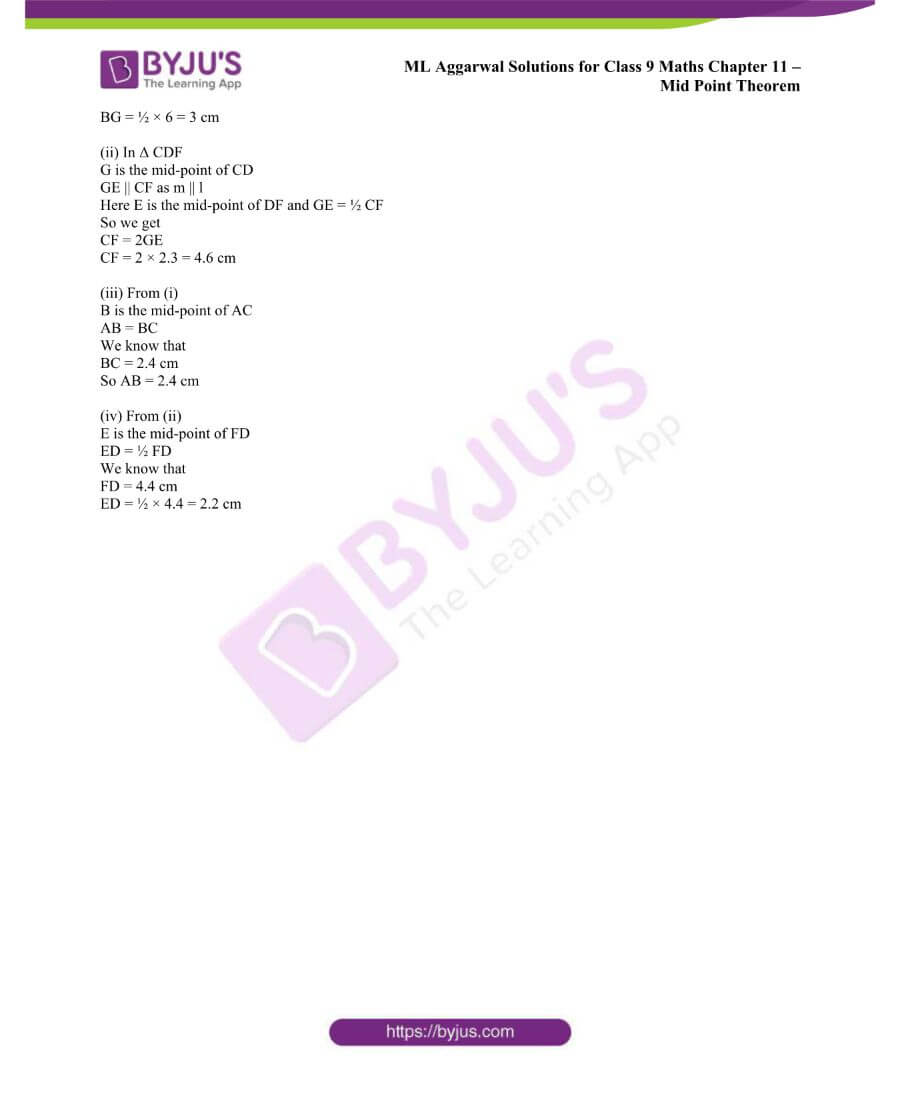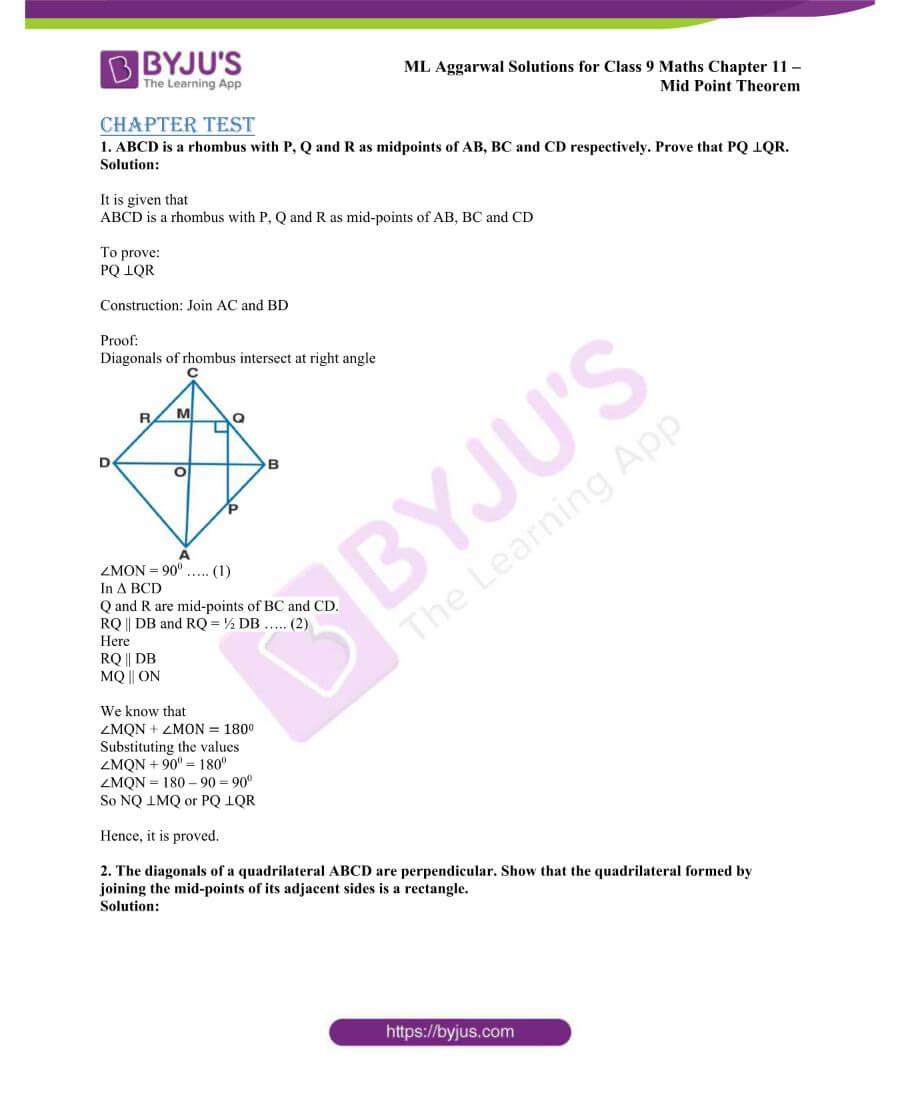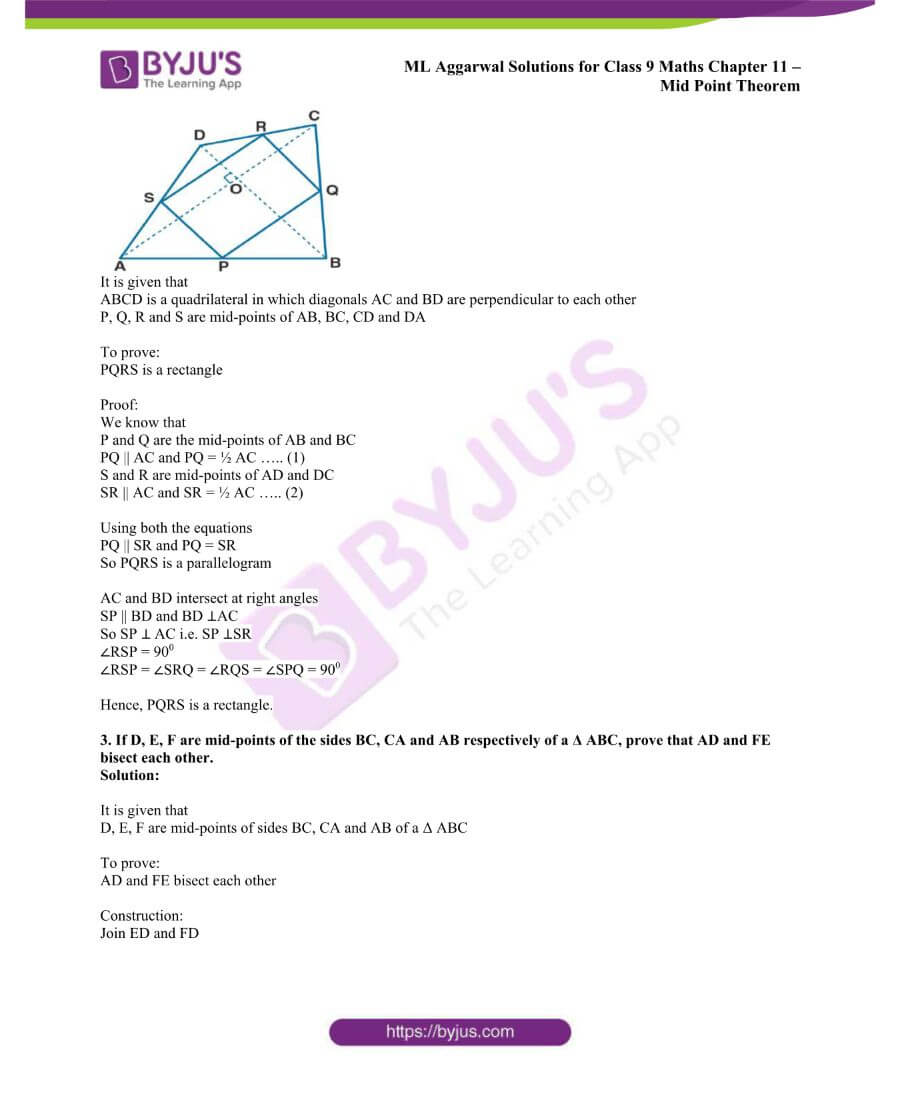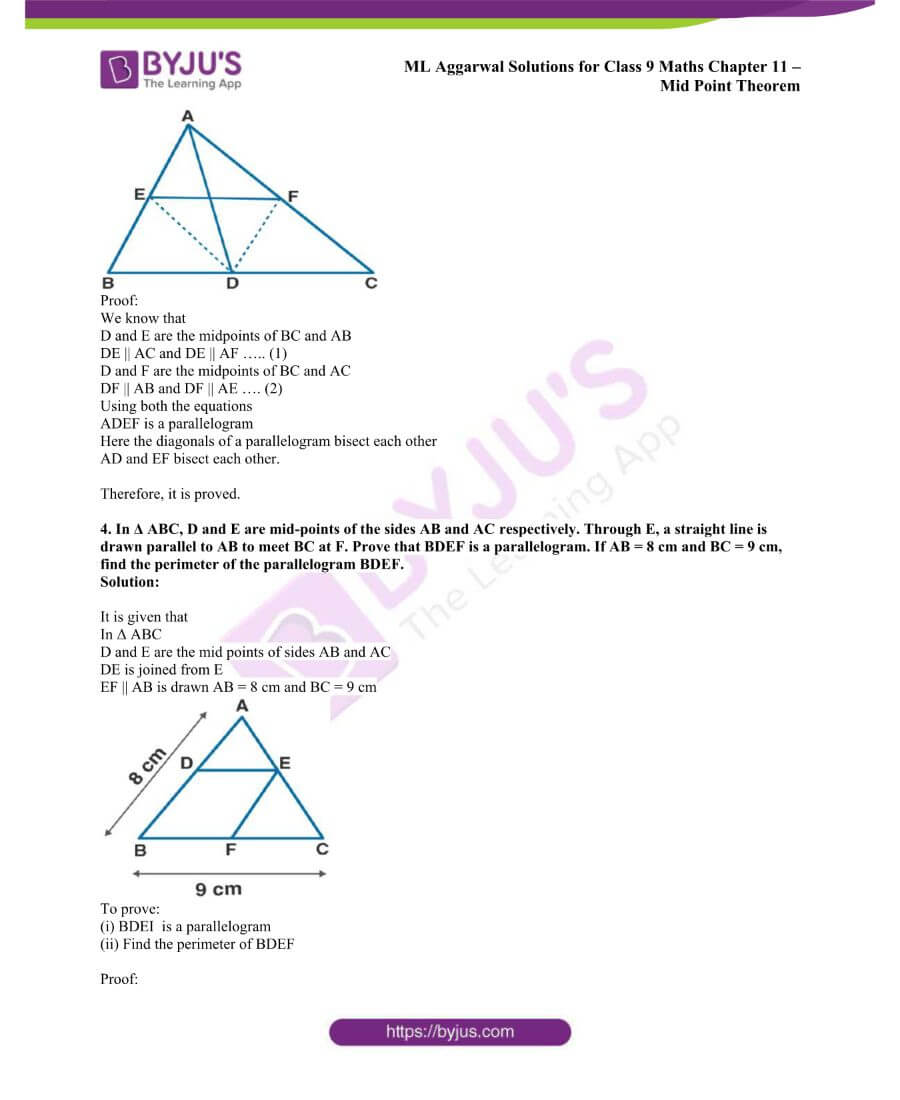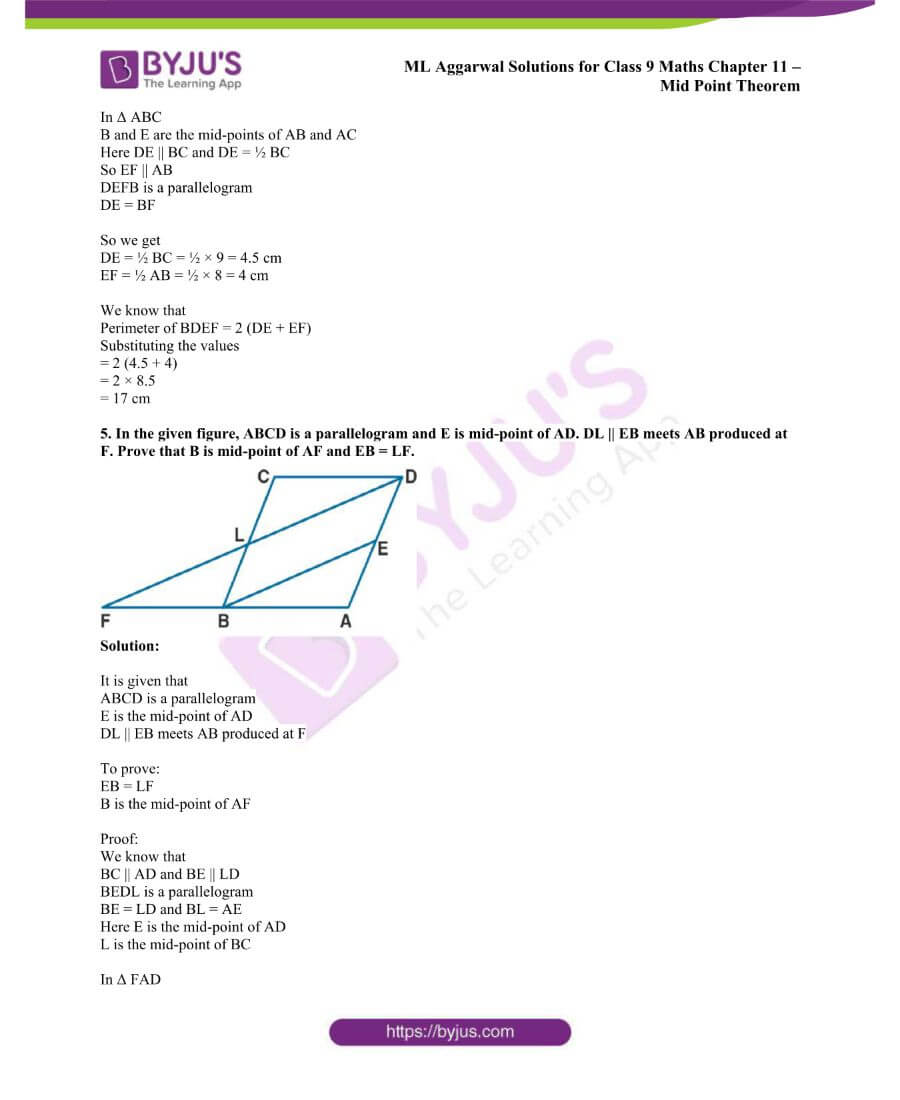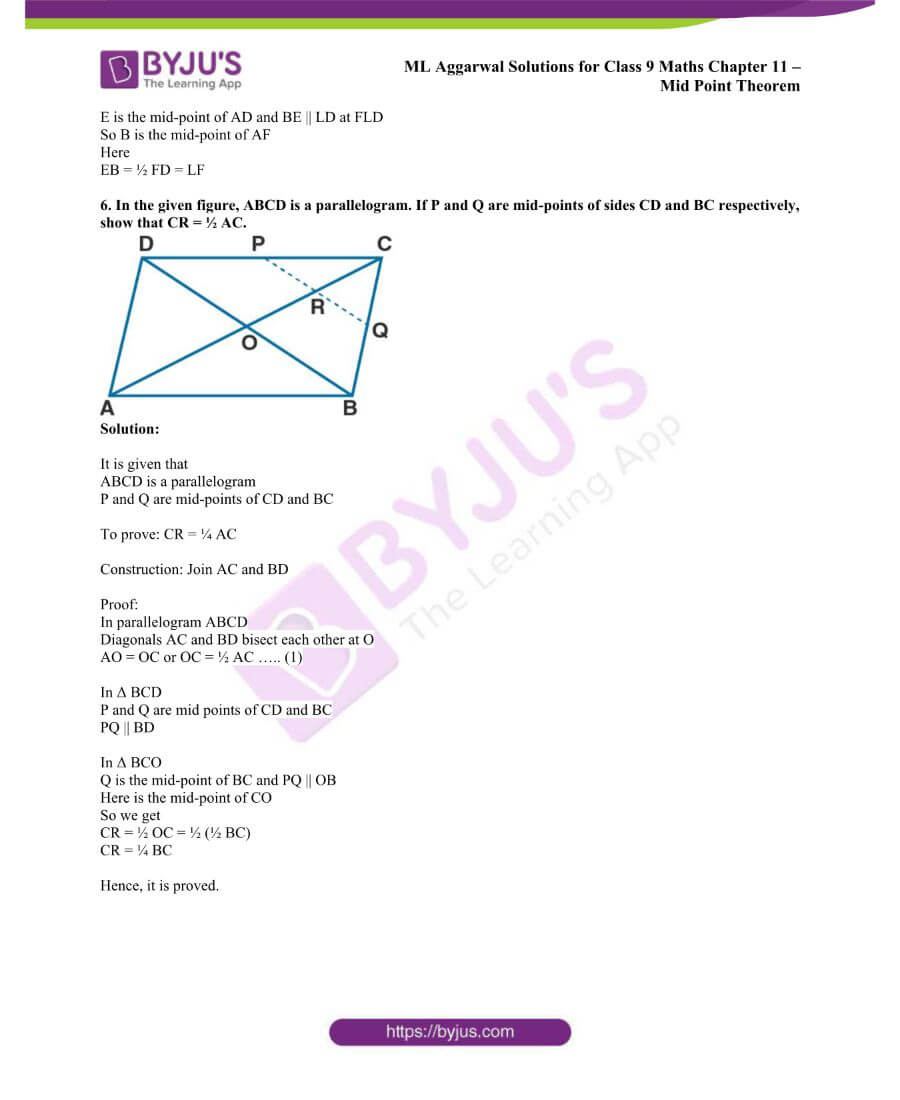### Access ML Aggarwal Solutions for Class 9 Maths Chapter 11: Mid Point Theorem

Exercise 11

1. (a) In the figure (1) given below, D, E and F are mid-points of the sides BC, CA and AB respectively of Δ ABC. If AB = 6 cm, BC = 4.8 cm and CA = 5.6 cm, find the perimeter of (i) the trapezium FBCE (ii) the triangle DEF.

(b) In the figure (2) given below, D and E are mid-points of the sides AB and AC respectively. If BC = 5.6 cm and ∠B = 720, compute (i) DE (ii) ∠ADE.

(c) In the figure (3) given below, D and E are mid-points of AB, BC respectively and DF || BC. Prove that DBEF is a parallelogram. Calculate AC if AF = 2.6 cm.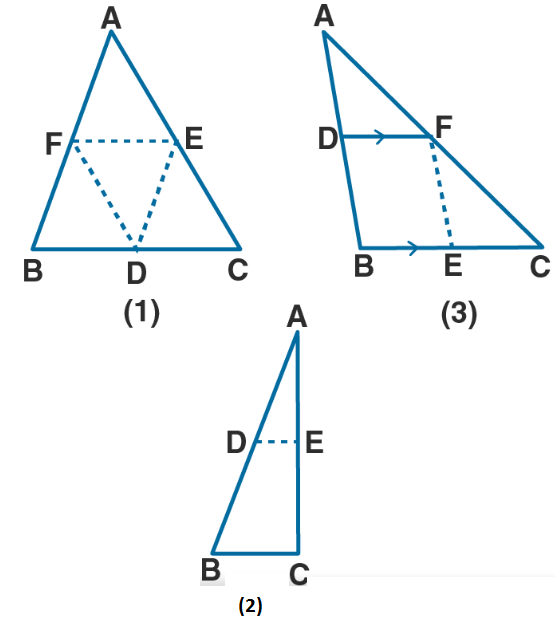Solution:

(a) (i) It is given that

AB = 6 cm, BC = 4.8 cm and CA = 5.6 cm

To find: The perimeter of trapezium FBCA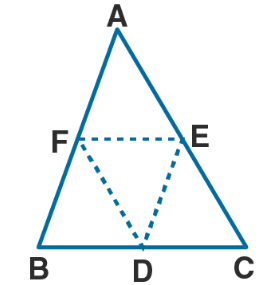It is given that

F is the mid-point of AB

We know that

BF = ½ AB = ½ × 6 = 3 cm ……. (1)

It is given that

E is the mid-point of AC

We know that

CE = ½ AC = ½ × 5.6 = 2.8 cm ……. (2)

Here F and E are the mid-point of AB and CA

FE || BC

We know that

FE = ½ BC = ½ × 4.8 = 2.4 cm …… (3)

Here

Perimeter of trapezium FBCE = BF + BC + CE + EF

Now substituting the value from all the equations

= 3 + 4.8 + 2.8 + 2.4

= 13 cm

Therefore, the perimeter of trapezium FBCE is 13 cm.

(ii) D, E and F are the midpoints of sides BC, CA and AB of Δ ABC

Here EF || BC

EF = ½ BC = ½ × 4.8 = 2.4 cm

DE = ½ AB = ½ × 6 = 3 cm

FD = ½ AC = ½ × 5.6 = 2.8 cm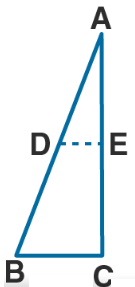We know that

Perimeter of Δ DEF = DE + EF + FD

Substituting the values

= 3 + 2.4 + 2.8

= 8.2 cm

(b) It is given that

D and E are the mid-point of sides AB and AC

BC = 5.6 cm and ∠B = 720

To find: (i) DE (ii) ∠ADE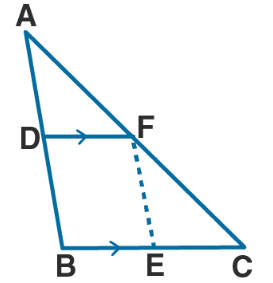We know that

In Δ ABC

D and E is the mid-point of the sides AB and AC

Using mid-point theorem

DE || BC

(i) DE = ½ BC = ½ × 5.6 = 2.8 cm

(ii) ∠ADE = ∠B are corresponding angles

It is given that

∠B = 720 and BC || DE

(c) It is given that

D and E are the midpoints of AB and BC respectively

DF || BC and AF = 2.6 cm

To find: (i) BEF is a parallelogram

(ii) Calculate the value of AC

Proof:

(i) In Δ ABC

D is the midpoint of AB and DF || BC

F is the midpoint of AC ….. (1)

F and E are the midpoints of AC and BC

EF || AB ….. (2)

Here DF || BC

DF || BE ….. (3)

Using equation (2)

EF || AB

EF || DB ….. (4)

Using equation (3) and (4)

DBEF is a parallelogram

(ii) F is the midpoint of AC

So we get

AC = 2 × AF = 2 × 2.6 = 5.2 cm

2. Prove that the four triangles formed by joining in pairs the mid-points of the sides C of a triangle are congruent to each other.

Solution:

It is given that

In Δ ABC

D, E and F are the mid-points of AB, BC and CA

Now join DE, EF and FD

To find:

Δ ADF ≅ Δ DBE ≅ Δ ECF ≅ Δ DEF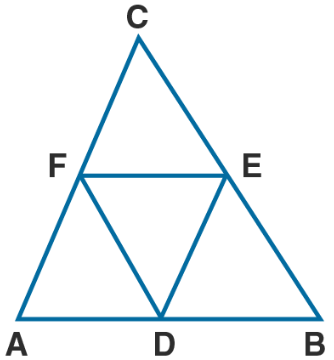To prove:

In Δ ABC

D and E are the mid-points of AB and BC

DE || AC or FC

Similarly DF || EC

DECF is a parallelogram

We know that

Diagonal FE divides the parallelogram DECF in two congruent triangles DEF and CEF

Δ DEF ≅ Δ ECF …… (1)

Here we can prove that

Δ DBE ≅ Δ DEF …. (2)

Δ DEF ≅ Δ ADF ……. (3)

Using equation (1), (2) and (3)

Δ ADF ≅ Δ DBE ≅ Δ ECF ≅ Δ DEF

3. If D, E and F are mid-points of sides AB, BC and CA respectively of an isosceles triangle ABC, prove that Δ DEF is also isosceles.

Solution:

It is given that

ABC is an isosceles triangle in which AB = AC

D, E and F are the midpoints of the sides BC, CA and AB

Now D, E and F are joined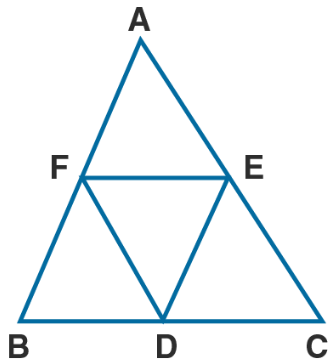To find:

Δ DEF is an isosceles triangle

Proof:

D and E are the midpoints of BC and AC

Here DE || AB and DE = ½ AB ….. (1)

D and F are the midpoints of BC and AB

Here DF || AC and DF = ½ AC ….. (2)

It is given that

AB = BC and DE = DF

Hence, Δ DEF is an isosceles triangle.

4. The diagonals AC and BD of a parallelogram ABCD intersect at O. If P is the midpoint of AD, prove that

(i) PQ || AB

(ii) PO = ½ CD.

Solution:

It is given that

ABCD is a parallelogram in which diagonals AC and BD intersect each other

At the point O, P is the midpoint of AD

Join OP

To find: (i) PQ || AB (ii) PQ = ½ CD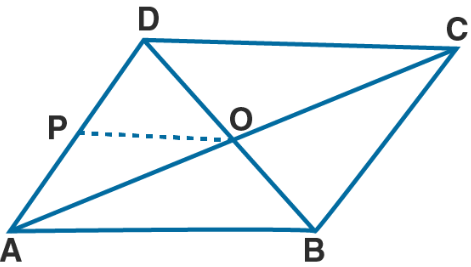Proof:

(i) In parallelogram diagonals bisect each other

BO = OD

Here O is the mid-point of BD

In Δ ABD

P and O is the midpoint of AD and BD

PO || AB and PO = ½ AB ….. (1)

Hence, it is proved that PO || AB.

(ii) ABCD is a parallelogram

AB = CD ……. (2)

Using both (1) and (2)

PO = ½ CD

5. In the adjoining figure, ABCD is a quadrilateral in which P, Q, R and S are mid-points of AB, BC, CD and DA respectively. AC is its diagonal. Show that

(i) SR || AC and SR = ½ AC

(ii) PQ = SR

(iii) PQRS is a parallelogram.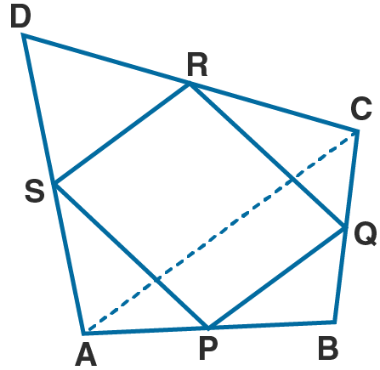Solution:

It is given that

P, Q, R and S are the mid-points of sides AB, BC, CD and DA

AC is the diagonal

To find:

(i) SR || AC and SR = ½ AC

(ii) PQ = SR

(iii) PQRS is a parallelogram

Proof:

S and R are the mid-points of AD and DC

SR || AC and SR = ½ AC….. (1) Using the mid-point theorem

(ii) In Δ ABC

P and Q are the midpoints of AB and BC

PQ || AC and PQ = ½ AC ….. (2)

Using equation (1) and (2)

PQ = SR and PQ || SR

(iii) PQ = SR and PQ || SR

Hence, PQRS is a parallelogram.

6. Show that the quadrilateral formed by joining the mid-points of the adjacent sides of a square, is also a square.

Solution:

It is given that

A square ABCD in which E, F, G and H are mid-points of AB, BC, CD and DA

Join EF, FG, GH and HE.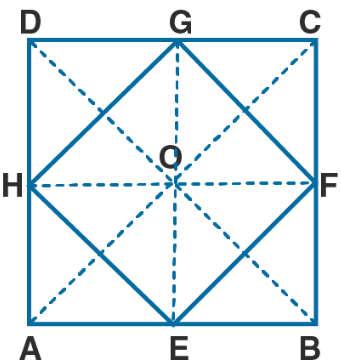To find:

EFGH is a square

Construct AC and BD

Proof:

In Δ ACD

G and H are the mid-points of CD and AC

GH || AC and GH = ½ AC ….. (1)

In Δ ABC, E and F are the mid-points of AB and BC

EF || AC and EF = ½ AC …… (2)

Using both the equations

EF || GH and EF = GH = ½ AC ….. (3)

In the same way we can prove that

EF || GH and EH = GF = ½ BD

We know that the diagonals of square are equal

AC = BD

By dividing both sides by 2

½ AC = ½ BD ….. (4)

Using equation (3) and (4)

EF = GH = EH = GF …. (5)

Therefore, EFGH is a parallelogram

In Δ GOH and Δ GOF

OH = OF as the diagonals of parallelogram bisect each other

OG = OG is common

Using equation (5)

GH = GF

Δ GOH ≅ Δ GOF (SSS axiom of congruency)

∠GOH = ∠GOF (c.p.c.t)

We know that

∠GOH + ∠GOF = 1800 as it is a linear pair

∠GOH + ∠GOH = 1800

So we get

2 ∠GOH = 1800

∠GOH = 1800/2 = 900

So the diagonals of a parallelogram ABCD bisect and perpendicular to each other

Hence, it is proved that EFGH is a square.

7. In the adjoining figure, AD and BE are medians of Δ ABC. If DF || BE, prove that CF = ¼ AC.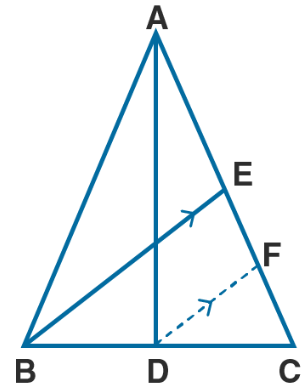Solution:

It is given that

AD and BE are the medians of Δ ABC

Construct DF || BE

To find:

CF = ¼ AC

Proof:

In Δ BCE

D is the mid-point of BC and DF || BE

F is the mid-point of EC

CF = ½ EC …… (1)

E is the mid-point of AC

EC = ½ AC ….. (2)

Using both the equations

CF = ½ EC = ½ (½ AC)

So we get

CF = ¼ AC

Hence, it is proved.

8. In the figure (1) given below, ABCD is a parallelogram. E and F are mid-points of the sides AB and CO respectively. The straight lines AF and BF meet the straight lines ED and EC in points G and H respectively. Prove that

(i) Δ HEB = Δ HCF

(ii) GEHF is a parallelogram.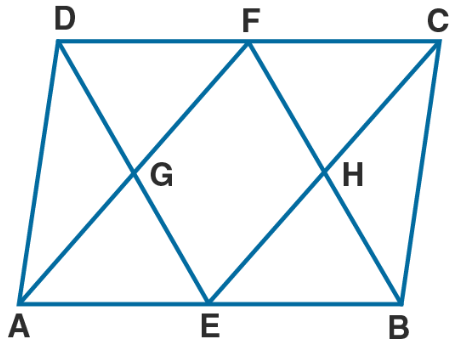Solution:

It is given that

ABCD is a parallelogram

E and F are the mid-points of sides AB and CD

To prove:

(i) Δ HEB = Δ HCF

(ii) GEHF is a parallelogram

Proof:

(i) We know that

ABCD is a parallelogram

FC || BE

∠CEB = ∠FCE are alternate angles

∠HEB = ∠FCH ….. (1)

∠EBF = ∠CFB are alternate angles

∠EBH = ∠CFM …… (2)

Here E and F are mid-points of AB and CD

BE = ½ AB ….. (3)

CF = ½ CD …… (4)

We know that

ABCD is a parallelogram

AB = CD

Now dividing both sides by ½

½ AB = ½ CD

Using equation (3) and (4)

BE = CF ….. (5)

In Δ HEB and Δ HCF

∠HEB = ∠FCH using equation (1)

∠EBH = ∠CFH using equation (2)

BE = CF using equation (5)

So we get

Δ HEB ≅ Δ HCF (ASA axiom of congruency)

Hence, it is proved.

(ii) It is given that

E and F are the mid-points of AB and CD

AB = CD

So we get

AE = CF

Here AE || CF

AE = CF and AE || CF

So AECF is a parallelogram.

G and H are the mid-points of AF and CE

GF || EH ……. (6)

In the same way we can prove that GFHE is a parallelogram

So G and H are the points on the line DE and BF

GE || HF …….. (7)

Using equation (6) and (7) GEHF is a parallelogram.

Hence, it is proved.

9. ABC is an isosceles triangle with AB = AC. D, E and F are mid-points of the sides BC, AB and AC respectively. Prove that the line segment AD is perpendicular to EF and is bisected by it.

Solution:

It is given that

ABC is an isosceles triangle with AB = AC

D, E and F are mid-points of the sides BC, AB and AC

To find:

AD is perpendicular to EF and is bisected by it.

Proof:

In Δ ABD and Δ ACD

ABC is an isosceles triangle

∠ABD = ∠ACD

Here D is the mid-point of BC

BD = BD

It is given that AB = AC

Δ ABD ≅ Δ ACD (SAS axiom of congruency)

∠ADB = ∠AOC (c. p. c. t)

We know that

∠ADB + ∠AOC = 1800 is a linear pair

By further calculation

So we get

So AD is perpendicular to BC ….. (1)

D and E are the mid-points of BC and AB

DE || AF …… (2)

D and F are the mid-points of BC and AC

Using equation (2) and (3)

AEDF is a parallelogram.

Here the diagonals of a parallelogram bisect each other

AD and EF bisect each other

Using equation (1) and (3)

EF || BC

So AD is perpendicular to EF

Hence, it is proved.

10. (a) In the quadrilateral (1) given below, AB || DC, E and F are the mid-points of AD and BD respectively. Prove that:

(i) G is mid-point of BC

(ii) EG = ½ (AB + DC)

(b) In the quadrilateral (2) given below, AB || DC || EG. If E is mid-point of AD prove that:

(i) G is the mid-point of BC

(ii) 2EG = AB + CD

(c) In the quadrilateral (3) given below, AB || DC.

E and F are mid-point of non-parallel sides AD and BC respectively. Calculate:

(i) EF if AB = 6 cm and DC = 4 cm.

(ii) AB if DC = 8 cm and EF = 9 cm.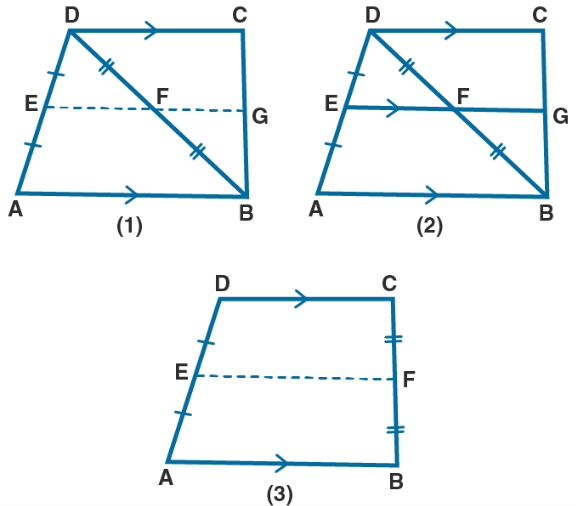Solution:

(a) It is given that

AB || DC, E and F are mid-points of AD and BD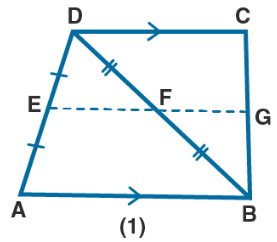To prove:

(i) G is mid-point of BC

(ii) EG = ½ (AB + DC)

Proof:

In Δ ABD

F is the mid-point of BD

DF = BF

E is the mid-point of AD

EF || AB and EF = ½ AB ….. (1)

It is given that AB || CD

EG || CD

F is the mid-point of BD

FG || DC

G is the mid-point of BC

FG = ½ DC …….. (2)

EF + FG = ½ AB + ½ DC

Taking ½ as common

EG = ½ (AB + DC)

Therefore, it is proved.

(b) It is given that

Quadrilateral ABCD in which AB || DC || EG

E is the mid-point of AD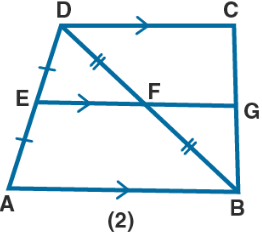To prove:

(i) G is the mid-point of BC

(ii) 2EG = AB + CD

Proof:

AB || DC

EG || AB

So we get

EG || DC

In Δ DAB,

E is the mid-point of BD and EF = ½ AB ….. (1)

In Δ BCD,

F is the mid-point of BD and FG || DC

FG = ½ CD …… (2)

EF + FG = ½ AB + ½ CD

Taking out the common terms

EG = ½ (AB + CD)

Hence, it is proved.

(c) It is given that

A quadrilateral in which AB || DC

E and F are the mid-points of non-parallel sides AD and BC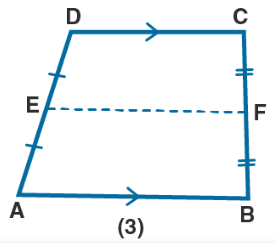To prove:

(i) EF if AB = 6 cm and DC = 4 cm.

(ii) AB if DC = 8 cm and EF = 9 cm.

Proof:

We know that

The length of line segment joining the mid-points of two non-parallel sides is half the sum of the lengths of the parallel sides

E and F are the mid-points of AD and BC

EF = ½ (AB + CD) …… (1)

(i) AB = 6 cm and DC = 4 cm

Substituting in equation (1)

EF = ½ (6 + 4)

By further calculation

EF = ½ × 10 = 5 cm

(ii) DC = 8 cm and EF = 9 cm

Substituting in equation (1)

EF = ½ (AB + DC)

By further calculation

9 = ½ (AB + 8)

18 = AB + 8

So we get

18 – 8 = AB

AB = 10 cm

11. (a) In the quadrilateral (1) given below, AD = BC, P, Q, R and S are mid-points of AB, BD, CD and AC respectively. Prove that PQRS is a rhombus.

(b) In the figure (2) given below, ABCD is a kite in which BC = CD, AB = AD, E, F, G are mid-points of CD, BC and AB respectively. Prove that:

(i) ∠EFG = 900

(ii) The line drawn through G and parallel to FE bisects DA.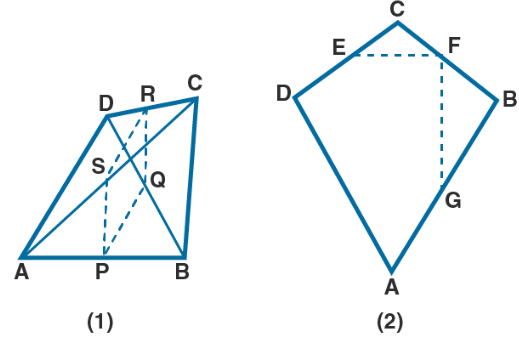Solution:

(a) It is given that

P, Q, R and S are mid-points of AB, BD, CD and AC

To prove:

PQRS is a rhombus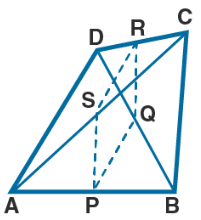Proof:

In Δ ABD

P and Q are mid points of AB and BD

PQ || AD and PQ = ½ AB …. (1)

In Δ BCD,

R and Q are mid points of DC and BD

RQ || BC and RQ = ½ BC …… (2)

P and S are mid-points of AB and AC

PS || BC and PS = ½ BC ……. (3)

Using all the equations

PS || RQ and PQ = PS = RQ

Here PS || RQ and PS = RQ

PQRS is a parallelogram

PQ = RS = PS = RQ

PQRS is a rhombus

Therefore, it is proved.

(ii) It is given that

ABCD is a kite in which BC = CD, AB = AD, E, F, G are mid-points of CD, BC and AB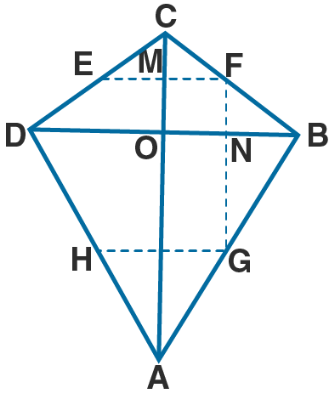To prove:

(i) ∠EFG = 900

(ii) The line drawn through G and parallel to FE bisects DA

Construction:

Join AC and BD

Construct GH through G parallel to FE

Proof:

(i) We know that

Diagonals of a kite interest at right angles

∠MON = 900 ….. (1)

In Δ BCD

E and F are mid-points of CD and BC

EF || DB and EF = ½ DB …… (2)

EF || DB

MF || ON

Here

∠MON + ∠MFN = 1800

900 + ∠MFN = 1800

By further calculation

∠MFN = 180 – 90 = 900

So ∠EFG = 900

Hence, it is proved.

(ii) In Δ ABD

G is the mid-point of AB and HG || DB

Using equation (2)

EF || DB and EF || HG

HG || DB

Here H is the mid-point of DA

Therefore, the line drawn through G and parallel to FE bisects DA.

12. In the adjoining figure, the lines l, m and n are parallel to each other, and G is mid-point of CD. Calculate:

(i) BG if AD = 6 cm

(ii) CF if GE = 2.3 cm

(iii) AB if BC = 2.4 cm

(iv) ED if FD = 4.4 cm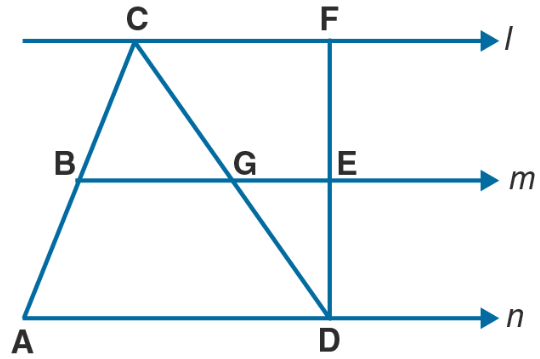Solution:

It is given that

The straight line l, m and n are parallel to each other

G is the mid-point of CD

To find:

(i) BG if AD = 6 cm

(ii) CF if GE = 2.3 cm

(iii) AB if BC = 2.4 cm

(iv) ED if FD = 4.4 cmProof:

(i) In Δ ACD,

G is the mid-point of CD

BG || AD as m || n

Here B is the mid-point of AC and BG = ½ AD

So we get

BG = ½ × 6 = 3 cm

(ii) In Δ CDF

G is the mid-point of CD

GE || CF as m || l

Here E is the mid-point of DF and GE = ½ CF

So we get

CF = 2GE

CF = 2 × 2.3 = 4.6 cm

(iii) From (i)

B is the mid-point of AC

AB = BC

We know that

BC = 2.4 cm

So AB = 2.4 cm

(iv) From (ii)

E is the mid-point of FD

ED = ½ FD

We know that

FD = 4.4 cm

ED = ½ × 4.4 = 2.2 cm

Chapter Test

1. ABCD is a rhombus with P, Q and R as midpoints of AB, BC and CD respectively. Prove that PQ ⊥QR.

Solution:

It is given that

ABCD is a rhombus with P, Q and R as mid-points of AB, BC and CD

To prove:

PQ ⊥QR

Construction: Join AC and BD

Proof:

Diagonals of rhombus intersect at right angle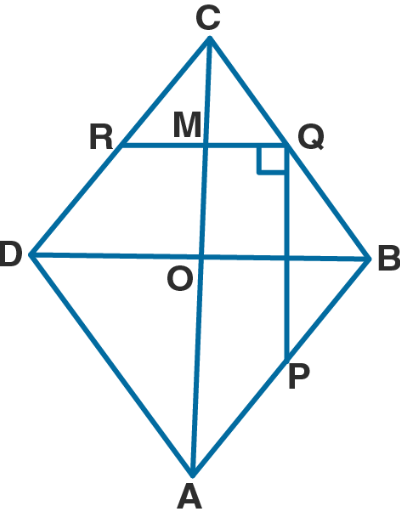∠MON = 900 ….. (1)

In Δ BCD

Q and R are mid-points of BC and CD.

RQ || DB and RQ = ½ DB ….. (2)

Here

RQ || DB

MQ || ON

We know that

∠MQN + ∠MON = 1800

Substituting the values

∠MQN + 900 = 1800

∠MQN = 180 – 90 = 900

So NQ ⊥MQ or PQ ⊥QR

Hence, it is proved.

2. The diagonals of a quadrilateral ABCD are perpendicular. Show that the quadrilateral formed by joining the mid-points of its adjacent sides is a rectangle.

Solution: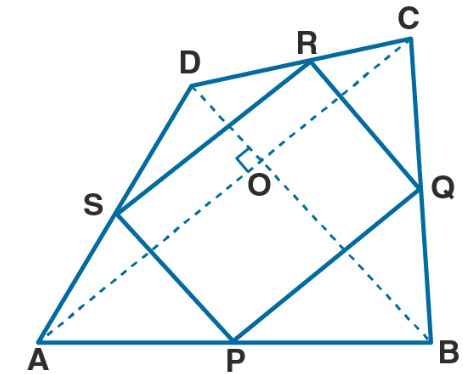It is given that

ABCD is a quadrilateral in which diagonals AC and BD are perpendicular to each other

P, Q, R and S are mid-points of AB, BC, CD and DA

To prove:

PQRS is a rectangle

Proof:

We know that

P and Q are the mid-points of AB and BC

PQ || AC and PQ = ½ AC ….. (1)

S and R are mid-points of AD and DC

SR || AC and SR = ½ AC ….. (2)

Using both the equations

PQ || SR and PQ = SR

So PQRS is a parallelogram

AC and BD intersect at right angles

SP || BD and BD ⊥AC

So SP ⊥ AC i.e. SP ⊥SR

∠RSP = 900

∠RSP = ∠SRQ = ∠RQS = ∠SPQ = 900

Hence, PQRS is a rectangle.

3. If D, E, F are mid-points of the sides BC, CA and AB respectively of a Δ ABC, prove that AD and FE bisect each other.

Solution:

It is given that

D, E, F are mid-points of sides BC, CA and AB of a Δ ABC

To prove:

AD and FE bisect each other

Construction:

Join ED and FD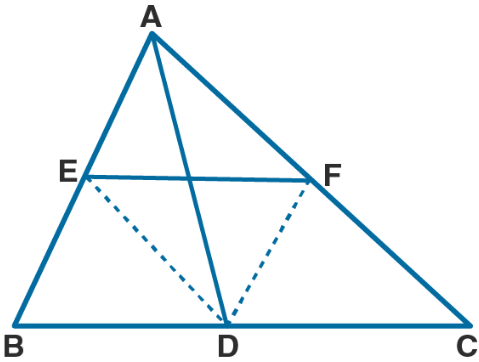Proof:

We know that

D and E are the midpoints of BC and AB

DE || AC and DE || AF ….. (1)

D and F are the midpoints of BC and AC

DF || AB and DF || AE …. (2)

Using both the equations

Here the diagonals of a parallelogram bisect each other

AD and EF bisect each other.

Therefore, it is proved.

4. In Δ ABC, D and E are mid-points of the sides AB and AC respectively. Through E, a straight line is drawn parallel to AB to meet BC at F. Prove that BDEF is a parallelogram. If AB = 8 cm and BC = 9 cm, find the perimeter of the parallelogram BDEF.

Solution:

It is given that

In Δ ABC

D and E are the mid points of sides AB and AC

DE is joined from E

EF || AB is drawn AB = 8 cm and BC = 9 cm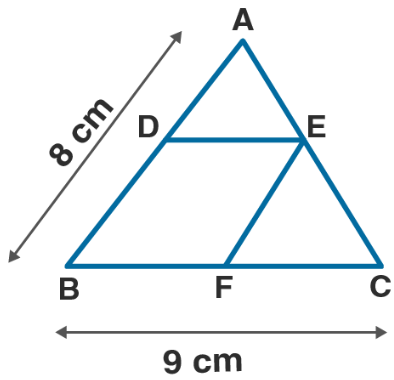To prove:

(i) BDEI is a parallelogram

(ii) Find the perimeter of BDEF

Proof:

In Δ ABC

B and E are the mid-points of AB and AC

Here DE || BC and DE = ½ BC

So EF || AB

DEFB is a parallelogram

DE = BF

So we get

DE = ½ BC = ½ × 9 = 4.5 cm

EF = ½ AB = ½ × 8 = 4 cm

We know that

Perimeter of BDEF = 2 (DE + EF)

Substituting the values

= 2 (4.5 + 4)

= 2 × 8.5

= 17 cm

5. In the given figure, ABCD is a parallelogram and E is mid-point of AD. DL || EB meets AB produced at F. Prove that B is mid-point of AF and EB = LF.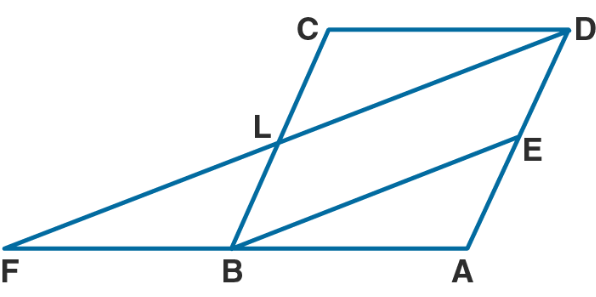Solution:

It is given that

ABCD is a parallelogram

E is the mid-point of AD

DL || EB meets AB produced at F

To prove:

EB = LF

B is the mid-point of AF

Proof:

We know that

BC || AD and BE || LD

BEDL is a parallelogram

BE = LD and BL = AE

Here E is the mid-point of AD

L is the mid-point of BC

E is the mid-point of AD and BE || LD at FLD

So B is the mid-point of AF

Here

EB = ½ FD = LF

6. In the given figure, ABCD is a parallelogram. If P and Q are mid-points of sides CD and BC respectively, show that CR = ½ AC.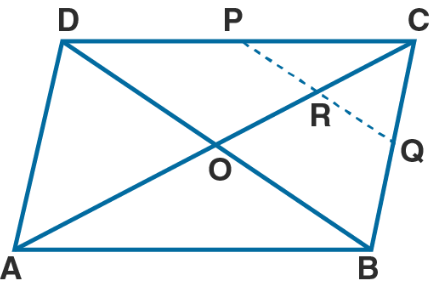Solution:

It is given that

ABCD is a parallelogram

P and Q are mid-points of CD and BC

To prove: CR = ¼ AC

Construction: Join AC and BD

Proof:

In parallelogram ABCD

Diagonals AC and BD bisect each other at O

AO = OC or OC = ½ AC ….. (1)

In Δ BCD

P and Q are mid points of CD and BC

PQ || BD

In Δ BCO

Q is the mid-point of BC and PQ || OB

Here is the mid-point of CO

So we get

CR = ½ OC = ½ (½ BC)

CR = ¼ BC

Hence, it is proved.